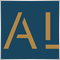# 为什么角度线的数据读不出来？54

double a=iMA(Symbol(),PERIOD_CURRENT,125,0,MODE_SMA,PRICE_CLOSE,200);
double b=iMA(Symbol(),PERIOD_CURRENT,125,0,MODE_SMA,PRICE_CLOSE,50);

ObjectCreate(0,"1",OBJ_TRENDBYANGLE,0,Time,a,Time,b);//画角度线

double d= ObjectGetDouble(0,"1",OBJPROP_PRICE,0);//价格
double e= ObjectGetDouble(0,"1",OBJPROP_PRICE,1);//价格
double f= ObjectGetDouble(0,"1",OBJPROP_ANGLE,2);//角度
Print("d=",d," e=",e," f=",f);45054673780654

Masonqq: